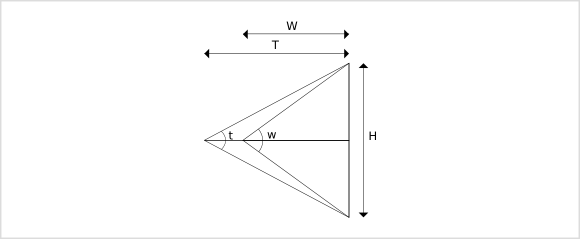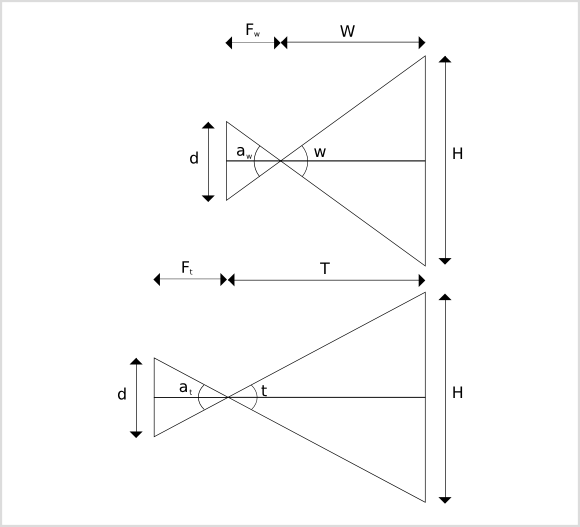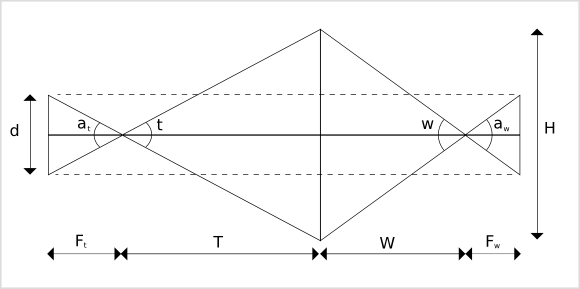## The Candy Theorem

#### February 20, 2010

I posted the Candy Theorem on the www.dpgr.gr forum on Febrary 17, 2010. This is a translation of the original post:

A friend recently bought a Nikon DX body. Discussing lenses, we concluded that it would be nice for him to have a 50mm lens: light, sharp, inexpensive, equivalent to a small telephoto (~75mm) as long as he owns the DX body, and a normal lens if he later upgrades to full frame. When he later wondered about the usability of a 35mm lens, I told him that the difference of a few mm can be matched with a few steps backwards.

Looking at the Nikon site (http://imaging.nikon.com/products/imaging/lineup/lens/simulator/index.htm) to visualize this difference (by changing the focal length in the third picture, it indeed gives the impression of stepping backwards), I got the idea that since the angles are known I can calculate precisely what “some steps backwards” means. This does not consist of any photographic matter of vital importance; it is just a simple and interesting exercise.

Disclaimer: w and t below do not refer to wide-angle and telephoto lenses in the strict sense; they are valid for any couple of different lenses. For example, between a 18mm and a 24mm lens, w is the 18mm and t is the 24mm. Between a 200mm and a 300mm lens, w is the 200mm and t is the 300mm.Therefore, if t is a telephoto lens angle of view and w is a wide-angle lens angle of view and the wide-angle lens sees height H at distance W, then there is a distance T which, if the telephoto lens is positioned at, it will also see height of H. Then

tan(t/2)=H/(2*T) <=> T=H/(2*tan(t/2))
tan(w/2)=H/(2*W) <=> W=H/(2*tan(w/2))
=>
T/W= (H/(2*tan(t/2)))/(H/(2*tan(w/2)))
<=>
T=W*tan(w/2)/tan(t/2)

For example, comparing a 35mm lens (63.33 degrees) to a 50mm lens (46.66 degrees)
T=W*tan(31.66)/tan(23.33)=W*1.43

…meaning you have to step 43% backwards to see with the 50mm lens what you see at a specific distance with the 35mm lens. If you shoot something at 10m, you have to step backwards another 4.3m.

15mm of focal length difference in the above example corresponds to 43% of difference in distance for the specific set of lenses, not for any given couple of lenses differing by 15mm. E.g, the 70mm and 85mm couple differ by approx 20%.

Btw, Nikon’s website provides the angles of view in degrees and minutes (1/60 of degree). If your calculator accepts degrees in decimal format, then X degrees + Y minutes = X + Y/60 degrees. To avoid miscalculation, do not forget that the formula uses the tangents of half the angles of view (w/2 , t/2).

UPDATE 17/2/2010 18:40

Looking into the relation between focal length and angle of view http://en.wikipedia.org/wiki/Angle_of_view

a/2 = arctan(d/(2*f))

…I came up with a second idea: why would I calculate the tangent of an angle which is provided by the arctangent related to the original variable (focal length) which I need to eventually compare? Two trigonometric functions and one division will result in 3 roundings. Not good.

The formula above refers to the triangle between the lens and the medium (film/sensor), which is similar (in the geometric sense) to the triangle between the lens and the object in the first diagram. In each case angle a is vertically opposite and thus equals w or t respectively. d/2 is the idol of H/2 on the sensor. The above results in the amazingly simple fact that

The ratio of the distances at which two different lenses capture the same-sized projection of a single object plane equals the ratio of their focal lengths.To prove it, let us define Fw as the focal length of the wide-angle lens and Ft of the telephoto lens. Then

w/2 = arctan(d/(2*Fw))
t/2 = arctan(d/(2*Ft))

And from the initial

T/W=tan(w/2)/tan(t/2) <=>
T/W=tan(arctan(d/(2*Fw)))/tan(arctan(d/(2*Ft))) <=>
T/W=(d/(2*Fw))/(d/(2*Ft)) <=>

T/W=Ft/Fw

beauty in simplicity… this is exciting!

In our example of 35mm to 50mm comparison:
T/W=50/35=1.43
In other words if I see an object of X width and Y height at 10m through the 35mm lens, I can see the same X width and Y height object through the 50mm lens if I step 4.3m backwards.

UPDATE 17/2/2010 22:20

I call it the Candy Theorem.

UPDATE 18/2/2010 9:55Proof B (easier)

Two isosceles triangles are similar if the angles between their congruent sides are equal (k), since their base angles (b) are b=(180-k)/2 …also equal.

Therefore, the isosceles triangle with congruent sides angle of at is similar to the one with angle of t. The same stands for the triangles with congruent sides angles of aw and w. Then

T/(H/2)=Ft/(d/2) <=> d/H=Ft/T
W/(H/2)=Fw/(d/2) <=> d/H=Fw/W
=>
Ft/T=Fw/W <=>
T/W=Ft/Fw

Obviously, the Candy Theorem works for steps forward as well.

In order for a 200mm lens to see the frame of a 300mm lens, one has to move forward
W/T=200/300=0.66

…by 33%.

In real life, of course, different lenses have different characteristics (sharpness, distortion, fall off, chromatic aberration, etc) and for each task there will always be a lens that does the job better than the others.

It is important to note that the Candy Theorem refers to a single frame, a single object plane of two dimensions: width and height, but no depth (or punctual depth to be precise). Moving forward or backwards will equalize one object plane, but if the image consists of more than one object plane, the other object planes will always appear disproportionate in the resulting two images recorded by two different lenses.

This is usually the case in wide to ultra-wide lenses, due to their inherent increased depth of field. With this in mind it is easier to visualize the focal length comparison of two telephoto lenses, since they are frequently used in single object plane photography (e.g. a portrait with blurred background).

A portrait viewed by a 105mm lens at 2.1m is viewed by a 135mm lens at 2.7m.